• t 5 print @cmdtext--发现多一个逗号下面逗号去掉 6 set @cmdtext=left(@cmdtext,len(@cmdtext)-2)--去掉逗号 7 set @cmdtext=@cmdtext+'from payphonemoney group by username' 8 print @cmdtext 9 exec(@cmdtext...

2017-08-03 回答

请注意转换数据库语言，再进行试验。

/*

普通行列转换

假设有张学生成绩表(tb)如下:

name subject result

张三 语文　　74

张三 数学　　83

张三 物理　　93

李四 语文　　74

李四 数学　　84

李四 物理　　94

*/

-------------------------------------------------------------------------

/*

想变成

姓名 语文 数学 物理

---------- ----------- ----------- -----------

李四 74 84 94

张三 74 83 93

*/

create table tb

(

[name] varchar(10) ,

subject varchar(10) ,

result int

)

insert into tb(name , subject , result) values('张三' , '语文' , 74)

insert into tb(name , subject , result) values('张三' , '数学' , 83)

insert into tb(name , subject , result) values('张三' , '物理' , 93)

insert into tb(name , subject , result) values('李四' , '语文' , 74)

insert into tb(name , subject , result) values('李四' , '数学' , 84)

insert into tb(name , subject , result) values('李四' , '物理' , 94)

go

--静态sql,指subject只有语文、数学、物理这三门课程。

select name 姓名,

max(case subject when '语文' then result else 0 end) 语文,

max(case subject when '数学' then result else 0 end) 数学,

max(case subject when '物理' then result else 0 end) 物理

from tb

group by name

/*

姓名 语文 数学 物理

---------- ----------- ----------- -----------

李四 74 84 94

张三 74 83 93

*/

--动态sql,指subject不止语文、数学、物理这三门课程。

declare @sql varchar(8000)

set @sql = 'select name as ' + '姓名'

select @sql = @sql + ' , max(case subject when ''' + subject + ''' then result else 0 end) [' + subject + ']'

from (select distinct subject from tb) as a

set @sql = @sql + ' from tb group by name'

exec(@sql)

/*

姓名 数学 物理 语文

---------- ----------- ----------- -----------

李四 84 94 74

张三 83 93 74

*/

-------------------------------------------------------------------

/*加个平均分，总分

姓名 语文 数学 物理 平均分 总分

---------- ----------- ----------- ----------- -------------------- -----------

李四 74 84 94 84.00 252

张三 74 83 93 83.33 250

*/

--静态sql,指subject只有语文、数学、物理这三门课程。

select name 姓名,

max(case subject when '语文' then result else 0 end) 语文,

max(case subject when '数学' then result else 0 end) 数学,

max(case subject when '物理' then result else 0 end) 物理,

cast(avg(result*1.0) as decimal(18,2)) 平均分,

sum(result) 总分

from tb

group by name

/*

姓名 语文 数学 物理 平均分 总分

---------- ----------- ----------- ----------- -------------------- -----------

李四 74 84 94 84.00 252

张三 74 83 93 83.33 250

*/

--动态sql,指subject不止语文、数学、物理这三门课程。

declare @sql1 varchar(8000)

set @sql1 = 'select name as ' + '姓名'

select @sql1 = @sql1 + ' , max(case subject when ''' + subject + ''' then result else 0 end) [' + subject + ']'

from (select distinct subject from tb) as a

set @sql1 = @sql1 + ' , cast(avg(result*1.0) as decimal(18,2)) 平均分,sum(result) 总分 from tb group by name'

exec(@sql1)

/*

姓名 数学 物理 语文 平均分 总分

---------- ----------- ----------- ----------- -------------------- -----------

李四 84 94 74 84.00 252

张三 83 93 74 83.33 250

*/

drop table tb

---------------------------------------------------------

---------------------------------------------------------

/*

如果上述两表互相换一下：即

姓名 语文 数学 物理

张三 74　　83　　93

李四 74　　84　　94

想变成

name subject result

---------- ------- -----------

李四 语文 74

李四 数学 84

李四 物理 94

张三 语文 74

张三 数学 83

张三 物理 93

*/

create table tb1

(

姓名 varchar(10) ,

语文 int ,

数学 int ,

物理 int

)

insert into tb1(姓名 , 语文 , 数学 , 物理) values('张三',74,83,93)

insert into tb1(姓名 , 语文 , 数学 , 物理) values('李四',74,84,94)

select * from

(

select 姓名 as name , subject = '语文' , result = 语文 from tb1

union all

select 姓名 as name , subject = '数学' , result = 数学 from tb1

union all

select 姓名 as name , subject = '物理' , result = 物理 from tb1

) t

order by name , case subject when '语文' then 1 when '数学' then 2 when '物理' then 3 when '总分' then 4 end

--------------------------------------------------------------------

/*加个平均分,总分

name subject result

---------- ------- --------------------

李四 语文 74.00

李四 数学 84.00

李四 物理 94.00

李四 平均分 84.00

李四 总分 252.00

张三 语文 74.00

张三 数学 83.00

张三 物理 93.00

张三 平均分 83.33

张三 总分 250.00

*/

select * from

(

select 姓名 as name , subject = '语文' , result = 语文 from tb1

union all

select 姓名 as name , subject = '数学' , result = 数学 from tb1

union all

select 姓名 as name , subject = '物理' , result = 物理 from tb1

union all

select 姓名 as name , subject = '平均分' , result = cast((语文 + 数学 + 物理)*1.0/3 as decimal(18,2)) from tb1

union all

select 姓名 as name , subject = '总分' , result = 语文 + 数学 + 物理 from tb1

) t

order by name , case subject when '语文' then 1 when '数学' then 2 when '物理' then 3 when '平均分' then 4 when '总分' then 5 end

drop table tb1

declare @cmdtext varchar(8000)

3 select @cmdtext=@cmdtext+' sum(case paytype when'''+paytype+'''then money else 0 end) as '''+paytype

4 +''','+char(10) from (select distinct paytype from payphonemoney) t

5 print @cmdtext--发现多一个逗号下面把逗号去掉

6 set @cmdtext=left(@cmdtext,len(@cmdtext)-2)--去掉逗号

7 set @cmdtext=@cmdtext+'from payphonemoney group by username'

8 print @cmdtext

9 exec(@cmdtext)

展开全文• excel怎么批量把行变成列

千次阅读 2020-12-22 13:30:13
1. excel中怎样批量将数据转换成数据你好，楼主想要的功能，可以使用VBA程序实现。(一)VBA程序代码如下：(代码运行之前请做好数据备份，以免丢失) Sub abcd()Dim i1, i2, i3, strOn Error Resume NextSet ...

1. excel中怎样批量将行数据转换成列数据

你好，楼主想要的功能，可以使用VBA程序实现。

(一)VBA程序代码如下：(代码运行之前请做好数据备份，以免丢失) Sub abcd()Dim i1, i2, i3, strOn Error Resume NextSet mysheet1 = ThisWorkbook.Worksheets("Sheet1") '定义工作表Sheet1For i1 = 1 To 1000 '从第1行到1000行 If mysheet1.Cells(i1, 1) <> "" Then '如果单元格不是空白，则 str = Mid(mysheet1.Cells(i1, 1), 1, 1) '截取第一个字符 If IsNumeric(str) = True Then '如果截取的字符是数字，则 i2 = i1 i3 = 1 Else i3 = i3 + 1 mysheet1.Cells(i2, i3) = mysheet1.Cells(i1, 1) '把ABCD项写入含有数字的那一行 End IfEnd IfNextEnd Sub (二)程序运行前后的结果如下图所示，望笑纳。 。

2. Excel 表格 批量将行数据换成列数据

可以使用公式来解决你的问题。

1、首先确认你的原始表格是从SHEET1的A1单元格开始排列的。

2、在sheet2的a1单元格中输入如下公式：

=IF(MOD(ROW(),3)=1,OFFSET(Sheet1!A$1,TRUNC(ROW()/3),0),IF(MOD(ROW(),3)=2,OFFSET(Sheet1!B$1,TRUNC(ROW()/3),0),IF(MOD(ROW(),3)=0,OFFSET(Sheet1!C$1,TRUNC(ROW()/3)-1,0),""))) 3、将此公式向下拖动，需要多少拖动多少。 任务完成 3. excel中怎么把多行多列转换成一行多列 参照此公式，在sheet2提取转换，在名称框(编辑栏左边，列标a上面)输入a1:av5(因，我只模拟了12列20行数据，所以提取过来的范围是a1:av5，你自己计算下应该是什么范围，用=address(1,360,4)可获取第360列(每一行是360个数据)第1行的单元格地址)回车，直接输入公式=INDEX(Sheet1!$A:$L,ROUNDUP(COLUMN(AV5)/12,0)+ROW(AV5)*30-30,MOD(COLUMN(AV5)-1,12)+1)同时按ctrl+回车结束。 4. EXCEL批量行转列(按图所示) 在J2:L2中分别输入或复制粘贴下列公式 =INDEX(A:A,MOD(ROW(A1)-1,5)+2) 【或=INDEX(A:A,MOD(ROW(A5),5)+2)】 =INDEX($1:$1,,INT((ROW(A1)-1)/5)+2) 【或=INDEX($1:$1,,INT(ROW(A10)/5))】 =INDEX($B$2:$F$6,MATCH(J2,$A$2:$A$6,),MATCH(K2,$B$1:$F$1，)) 选择J2:L2，下拉填充 如果A不止5行，将J2K2公式中的5修改为相应的行数。 5. EXCEL 如何把多列多行转换成一列多行 1. 需要将A列到C列的数值复制到一列中。 2. 点击“剪贴板”右下角的小按钮，在页面左面弹出了剪贴板对话框。 3. 拖动鼠标左键选中A2:C5单元格区域，点击鼠标右键，在弹出的菜单中选择“复制”选项。 4. 双击F2单元格，然后点击粘帖板中复制的内容，将内容复制到F2单元格中。 5. 将光标移动到公式编辑栏中，选中数据内容，点击鼠标右键，弹出的菜单中选择“复制”选项。 6. 然后按“ESC”键，按“CTUL+V”组合键将数据内容重新复制到F2单元格。 7. 调整列宽，依次点击【开始】-【填充】-【两端对齐】选项。 8. 弹出“Mircrosoft Office Excel”对话框，点击【确定】按钮。 9. 可以看到数值就被复制过来了且在一列中列式。此时，数值是文本格式，点击小按钮，在弹出的选项中选择“转换为数字”选项，这样文本格式的内容就转换成了数值形式。 6. excel怎麽批量把横排数据变成一列 单看你的例子 方法很多 假设你的数据在A1:J1 最快捷的基本操作 复制原数据，右击目的地单元格，选择性粘贴，转置，确定 公式的话 =OFFSET(A$1,,ROW(A1)-1)

=INDEX($1:$1,ROW(1:1))

=INDIRECT(CHAR(64+ROW(1:1))&1)

往下拖拉填充

=TRANSPOSE(A1:J1)

多单元格数组公式

7. 如何把excel多列(每列不同行),变成一列

可以使用如下宏代码实现操作目的。

Sub ComCol()

On Error Resume Next

Dim i As Single

Dim Fcol, Ecol As Single

Dim myRange As Range

Set myRange = Application.InputBox("选择区域"， Type:=8)

For i = Fcol + 1 To Ecol

Range(Cells(1, i), Cells(Cells(65535, i).End(xlUp).Row, i)).Copy Destination:=Cells(Cells(65535, Fcol).End(xlUp).Row + 1, Fcol)

Next

End Sub展开全文• 在日常取数或者数据处理中，我们会遇到需要便多行的数据拆分操作，或者时需要多行的合并操作，接下来，就总结下在hive中通过lateral view explode和concat/concat_ws的处理方法。 一列变多行lateral ...

在日常取数或者数据处理中，我们会遇到需要把一列变多行的数据拆分操作，或者是需要把多行变一列的合并操作，接下来，就总结下在sql中通过lateral view explode()和concat_ws()/collect_set()的处理方法。

一列变多行lateral view explode()

假设有这样一个 表1（table_1）

idtag
43fjkdljtag1|tag2|tag6
757jhidftag3|tag5
863hskdhtag1|tag9|tag10|tag11

从表中可以看出一个id对应的tag标签有多个，想要把它拆分开，变成以下 表2（table_2）

idtag
43fjkdljtag1
43fjkdljtag2
43fjkdljtag6
757jhidftag3
757jhidftag5
863hskdhtag1
863hskdhtag9
863hskdhtag10
863hskdhtag11

那么就可以使用lateral view explode()实现：

select id
,tag_1
from   table_1 lateral view explode(split(tag,'\\|'))tag as tag_1

上述语句中explode()里可以说明分隔符是什么，对于"|"注意转义符，所以是“\\|”

多行变一列concat_ws()/collect_set()

但如果是上述表2要转换成表1的格式，就需要做合并操作，实现如下：

select id
,concat_ws('\\|',collect_set(tag)) as tag
from table_2
group by id

concat_ws()可以根据指定连接符，上述语句也可以使用“-”连接，看自己需要和方便极客，要强调的是合并操作使用collect_set()，最后，不要忘记group by哦！

好了，就到这里吧，有问题欢迎大家留言评论~

展开全文hive sql
• 准备数据：CREATE TABLEtb_score(idINT(11) NOT NULLauto_increment,useridVARCHAR(20) NOT NULL COMMENT '用户id',subjectVARCHAR(20) COMMENT '科目',scoreDOUBLE COMMENT '成绩',PRIMARY KEY(id))ENGINE= ...

行转列

准备数据：

CREATE TABLEtb_score(

idINT(11) NOT NULLauto_increment,

useridVARCHAR(20) NOT NULL COMMENT '用户id',

subjectVARCHAR(20) COMMENT '科目',

scoreDOUBLE COMMENT '成绩',PRIMARY KEY(id)

)ENGINE= INNODB DEFAULT CHARSET =utf8;INSERT INTO tb_score(userid,subject,score) VALUES ('001','语文',90);INSERT INTO tb_score(userid,subject,score) VALUES ('001','数学',92);INSERT INTO tb_score(userid,subject,score) VALUES ('001','英语',80);INSERT INTO tb_score(userid,subject,score) VALUES ('002','语文',88);INSERT INTO tb_score(userid,subject,score) VALUES ('002','数学',90);INSERT INTO tb_score(userid,subject,score) VALUES ('002','英语',75.5);INSERT INTO tb_score(userid,subject,score) VALUES ('003','语文',70);INSERT INTO tb_score(userid,subject,score) VALUES ('003','数学',85);INSERT INTO tb_score(userid,subject,score) VALUES ('003','英语',90);INSERT INTO tb_score(userid,subject,score) VALUES ('003','政治',82);

查询数据表中的内容：

SELECT * FROM tb_score;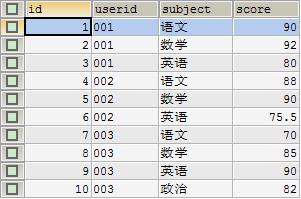如果我们需要将 subject 字段的多行内容选出来，作为结果集中的不同列，并根据 userid 进行分组显示对应的score，怎么实现呢？

使用 case...when....then 进行行转列

SELECTuserid,SUM(CASE subject WHEN '语文' THEN score ELSE 0 END) AS '语文',SUM(CASE subject WHEN '数学' THEN score ELSE 0 END) AS '数学',SUM(CASE subject WHEN '英语' THEN score ELSE 0 END) AS '英语',SUM(CASE subject WHEN '政治' THEN score ELSE 0 END) AS '政治'

FROMtb_scoreGROUP BY userid;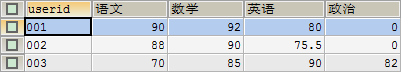使用 IF() 进行行转列

SELECTuserid,SUM(IF(subject='语文',score,0)) AS '语文',SUM(IF(subject='数学',score,0)) AS '数学',SUM(IF(subject='英语',score,0)) AS '英语',SUM(IF(subject='政治',score,0)) AS '政治'

FROMtb_scoreGROUP BY userid;注意点：SUM() 是为了能够使用 GROUP BY 根据 userid 进行分组，因为每一个 userid 对应的 subject="语文" 的记录只有一条，所以 SUM() 的值就等于对应那一条记录的 score 的值。

使用 SUM(IF()) 生成列 + WITH ROLLUP + 子查询生成汇总行

SELECT IFNULL(userid,'total') ASuserid,SUM(IF(subject='语文',score,0)) AS语文,SUM(IF(subject='数学',score,0)) AS数学,SUM(IF(subject='英语',score,0)) AS英语,SUM(IF(subject='政治',score,0)) AS政治,SUM(IF(subject='total',score,0)) AStotalFROM(SELECT userid,IFNULL(subject,'total') AS subject,SUM(score) ASscoreFROMtb_scoreGROUP BYuserid,subjectWITHROLLUPHAVING userid IS NOT NULL)ASAGROUP BYuseridWITH ROLLUP;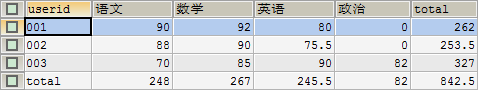使用 SUM(IF()) 生成列 + UNION 生成汇总行

SELECTuserid,SUM(IF(subject = '语文', score, 0)) AS语文,SUM(IF(subject = '数学', score, 0)) AS数学,SUM(IF(subject = '英语', score, 0)) AS英语,SUM(IF(subject = '政治', score, 0)) AS政治,SUM(score) ASTOTALFROMtb_scoreGROUP BYuseridUNION

SELECT

'TOTAL',SUM(IF(subject = '语文', score, 0)) AS语文,SUM(IF(subject = '数学', score, 0)) AS数学,SUM(IF(subject = '英语', score, 0)) AS英语,SUM(IF(subject = '政治', score, 0)) AS政治,SUM(score)FROM tb_score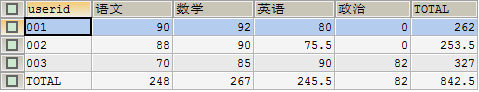使用 SUM(IF()) 生成列 + WITH ROLLUP 生成汇总行

SELECTIFNULL(userid,'TOTAL') ASuserid,SUM(IF(subject = '语文', score, 0)) AS语文,SUM(IF(subject = '数学', score, 0)) AS数学,SUM(IF(subject = '英语', score, 0)) AS英语,SUM(IF(subject = '政治', score, 0)) AS政治,SUM(score) ASTOTALFROMtb_scoreGROUP BY userid WITH ROLLUP ;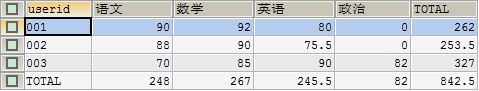使用 group_concat() 合并字段显示

SELECTuserid,

GROUP_CONCAT(subject, ":", score)AS成绩FROMtb_scoreGROUP BY userid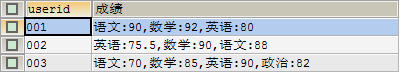列转行

准备数据：

CREATE TABLEtb_score1(

idINT(11) NOT NULLauto_increment,

useridVARCHAR(20) NOT NULL COMMENT '用户id',

cn_scoreDOUBLE COMMENT '语文成绩',

math_scoreDOUBLE COMMENT '数学成绩',

en_scoreDOUBLE COMMENT '英语成绩',

po_scoreDOUBLE COMMENT '政治成绩',PRIMARY KEY(id)

)ENGINE= INNODB DEFAULT CHARSET =utf8;INSERT INTO tb_score1(userid,cn_score,math_score,en_score,po_score) VALUES ('001',90,92,80,0);INSERT INTO tb_score1(userid,cn_score,math_score,en_score,po_score) VALUES ('002',88,90,75.5,0);INSERT INTO tb_score1(userid,cn_score,math_score,en_score,po_score) VALUES ('003',70,85,90,82);

查询数据表中的内容：

SELECT * FROM tb_score1;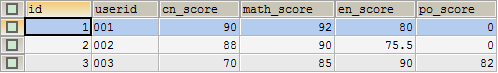如果我们需要将 userid 的每个科目分数分散成一条记录显示出来，怎么实现呢？

SELECT userid,'语文' AS course,cn_score AS score FROMtb_score1UNION ALL

SELECT userid,'数学' AS course,math_score AS score FROMtb_score1UNION ALL

SELECT userid,'英语' AS course,en_score AS score FROMtb_score1UNION ALL

SELECT userid,'政治' AS course,po_score AS score FROMtb_score1ORDER BY userid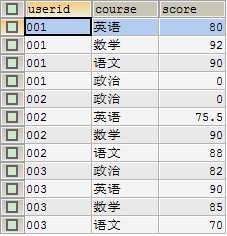这里将每个 userid 对应的多个科目的成绩查出来，通过 UNION ALL 将结果集加起来，达到上图的效果。

展开全文mysql列变行显示
• 可以将我的页面拆分成一个顶部(50％高度，宽度= 100％)和...将html页面拆分为CSS devide window into three (horizontal, 2 vertical).wrapM {width: 100%;height: 100%x;padding:2px;}.wrapT {width: 100%;h...
• 今天有个需求，要将数据透视表的二级转换为进行展示。具体如图： 数据透视表为这样： 最终效果为： 操作如下： 第一，将数据透视表改成表格格式，复制到新的表格里 2. CRTL+G 定位空值，输入"a...excel
• 现在,您需要将旧表转置到新表中： var maxColumns = 0; // Find the max number of columns for(var r = 0; r ; r++) { if(myTable.rows[r].cells.length > maxColumns) { maxColumns = myTable.rows[r].cells....
• 选中并复制需要转换的数据 在需要填充数据的单元格中单击右键，选择“选择性粘贴”菜单 在打开的“选择性粘贴”对话框中，勾选“转置” ４.　点击“确定”按钮，即可完成转换，数据转换为数据同理 ...excel
• Oracle转行，oracle下可以用函数decode处理:select 产品名称,sum(decode(季度,'第一季度',销售额,0)) 第一季度销售额,sum(decode(季度,'第二季度',销售额,0)) 第二季度销售额,sum(decode(季度,'第三季度',...
• --pivot(专列，将多行的值改为多显示)(for in的那个column,是某个的值，也就是将某个的值作为新的的column,这个column下边的值好像只能来自一) select * from(select risk.risk_id, re.risk_area_order,...
• 现我们准备它转为多。表格里数据除掉标题行行，总共有60数据，干脆我们就给它转为106吧。那么，一转多行多，怎么转换才快速呢？动手前，我们先找找规律吧。首先，我们要知道，表格单元格数据区域为A2:...
• oracle SQL竖表转横表()T_T_STUDENT表查询记录如下，要转成横表姓名 课程 成绩1 张飞 语文 802 张飞 数学 873 关羽 语文 974 张飞 英语 685 关羽 数学 536 刘备 语文 90create t...
• 一、转行 1、背景描述 在日常处理数据过程中，你们可能会经常遇到这种类型的数据： 而我们用pandas进行统计分析时，往往需要将结果转换成以下类型的数据： 2.方法描述 准备数据 df = pd.DataFrame({'姓名': ['A'...python pandas 数据处理 数据分析
• 原表数据:姓名 科目 分数张三 语文 100张三 数学 200张三 英语 300李四 语文 600李四 数学 700李四 英语 800转换后:姓名 语文 数学 英语张三 100 200 300李四 600 700 800实现方法：with cuspoint as(select '张三' ...
• 查询某个表(如证书表)，里面一个人一个证书就是一数据，一个人可能有多个证书(即多行)。需要显示为某个人所有证书显示在一，用标识符隔开。如下：要得结果为：SQL语句如下：SELECTpk_psndoc,LISTAGG( to_char...
• 数据库结构如图：而我想让同一个人的不同成绩变成此人在这一不同上显示出来，此时分为2中展现：第一种展现如图----【多行】(合并后的数据在同一上)：sql如下：select name ,group_concat(sore Separator...
• {"moduleinfo":{"card_count":[{"count_phone":1,"count":1}],"search_count":[{"count_phone":4,"count":4}]},"card":[{"des":"阿里云数据库专家保驾护航，为用户的数据库应用系统进行性能和风险评估，参与配合进行...
• 楔子笔者曾经碰到过两种格式的数据，当时确实我难住了，最后虽然解决了，但是方法不够优雅，而且效率也不高，如果想高效率，那么就必须使用pandas提供的方法。而pandas作为很强的一个库，一定可以优雅地解决。当时...
• --listagg(合并多行的值为字符串，只用一来显示)select status, count(*), listagg(risk_id, ',') within group (order by risk_id) from rp_risk group by status;select risk_id, status, listagg(risk_id, ',')...
• shell 行转列

2021-05-18 05:45:30
shell 源文本如下：a1 a2 a31 2 311 22 33输出的文本：a1:1a1:11a2:2a2:22a3:3a3:33命令：[root@SourceGG106 rkdir]# echo '> a1 a2 a3> 1 2 3> 11 22 33> ' |awk -F'' '{if(NF>3){print \$3}}'...
• 需求：将“项目名称”下的数据 ，横向变成列，然后根据“患者ID”，在对应的填对应的值，没有值的不用填。最终每一的数据，包含一个“患者ID”的数据。 完整代码： import pandas as pd df_org = pd.DataFrame...python
• http://blog.csdn.net/rainyspring4540/article/details/50231435这里是oracle10g的环境数据库结构如下： 如果我想将相同名字的成绩显示在同一，有2中基本展示：第一种展示(显示在同一的不同上) 其sql如下：...
• insert into #hbtab select name,(orderdate+'_banjie') as [year],comnum,orderdate from #test select * from #hbtab --获取的属性 --select *from #hbtab select @event=@event+',['+[year]+']' from (select ...java 动态行转列
• SELECT GROUP_CONCAT( CONCAT(’"’,id,’"’) separator ‘,’) from dual
• sql语句?怎么转啊select MIN(id) as ID, name, sex,sum(case when num=2 then 2 end) as num2,sum(case when num=3 then 3 end) as num3,sum(case when num=4 then 4 end) as num4,sum(case when num=5 then 5...
• MySQL中的any_value() 与 group_concat()函数 any_value() 会选择被分到同一组的数据里第一条数据的指定值作为返回数据，和老版本的MySQL默认是同一...左右：分组时数据压缩到一，用指定的分隔符分隔。 ...sql 数据库 mysql
• np.newaxis 新增一个轴如何将数组[0,1,2]转换成向量用ndarray[: , np.newaxis]代码实质就是将原本的(0,1,2)移到上，然后新增一其实可以更简单ndarray.shape=(3,1)>> x = np.arange(3)>> xarray([0...
• 行變SQL> select * from a3;ID ID1 ID2---------- ---------- ----------1 2 3SQL>SQL> select decode(column_name,column_name,column_name) name,2 decode(col......